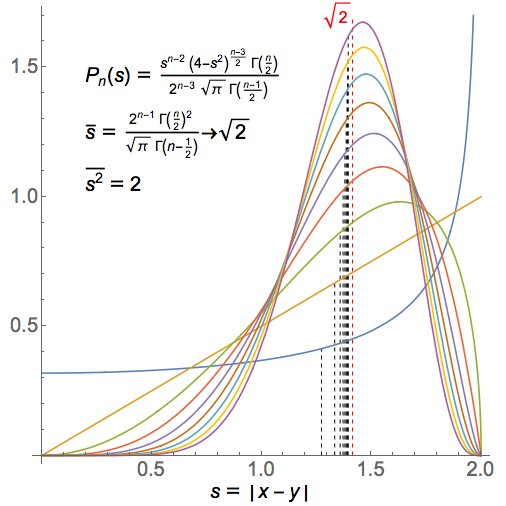Egan showed that this was indeed true:He wrote:

> Here’s the general formula for the distribution, with plots for n=2,...,10. The mean distance does tend to √2, and the mean of the squared distance is always exactly 2, so the variance tends to zero.